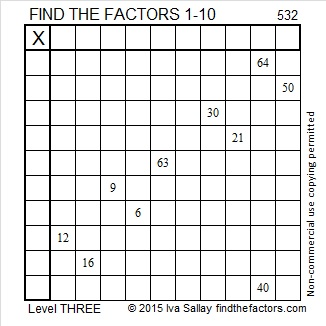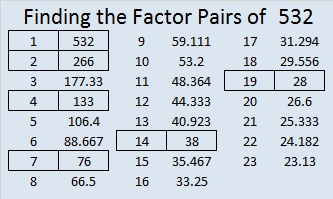# 532 and Level 3

532 is the sum of consecutive prime numbers 263 and 269.

532 is also the 19th pentagonal number.Print the puzzles or type the solution on this excel file: 10 Factors 2015-06-22

—————————————————————————————————

• 532 is a composite number.
• Prime factorization: 532 = 2 x 2 x 7 x 19, which can be written 532 = (2^2) x 7 x 19
• The exponents in the prime factorization are 1, 2, and 1. Adding one to each and multiplying we get (2 + 1)(1 + 1)(1 + 1) = 3 x 2 x 2 = 12. Therefore 532 has exactly 12 factors.
• Factors of 532: 1, 2, 4, 7, 14, 19, 28, 38, 76, 133, 266, 532
• Factor pairs: 532 = 1 x 532, 2 x 266, 4 x 133, 7 x 76, 14 x 38, or 19 x 28
• Taking the factor pair with the largest square number factor, we get √532 = (√4)(√133) = 2√133 ≈ 23.065125—————————————————————————————————

A Logical Approach to solve a FIND THE FACTORS puzzle: Find the column or row with two clues and find their common factor. Write the corresponding factors in the factor column (1st column) and factor row (top row).  Because this is a level three puzzle, you have now written a factor at the top of the factor column. Continue to work from the top of the factor column to the bottom, finding factors and filling in the factor column and the factor row one cell at a time as you go.This site uses Akismet to reduce spam. Learn how your comment data is processed.